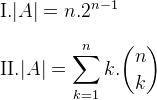# GATE | GATE CS 2019 | Question 20

Let U = {1, 2, …,n} and A = {(x, X)∣ x∈X, X⊆U }. Consider the following two statements on ∣A∣.Which of the above statements is/are TRUE?
(A) Only I
(B) Only II
(C) Both I and II
(D) Neither I nor II

Explanation: Given, A = {(x, X)∣ x∈X, X⊆U }, where U = {1, 2, …,n}.

As we know that The number of k element subsets of a set U with n elements = nCk.

The number of possible ordered pairs (x, X) where x ∈ X is k⋅ nCk for a given value of k from 1 to n. So total number of ordered pairs in A,So, both given statements are correct.

Option (C) is true.

Quiz of this Question

My Personal Notes arrow_drop_up
Article Tags :

Be the First to upvote.

Please write to us at contribute@geeksforgeeks.org to report any issue with the above content.